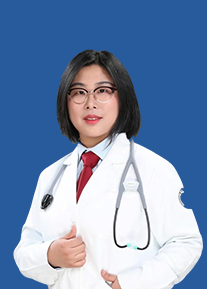• 咨询医生
• 体检预约

• 省直
• 杭州市
• 衢州市
• 湖州市
• 嘉兴市
• 宁波市
• 绍兴市
• 台州市
• 温州市
• 丽水市
• 金华市
• 舟山市
• 义乌市

• 浙江省中医院
• 浙江省人民医院
• 浙江大学医学院附属第一医院
• 浙江大学医学院附属第二医院
• 浙江大学医学院附属妇产科医院
• 浙江大学医学院附属邵逸夫医院
• 浙江大学儿童医院(湖滨老院区)
• 浙江大学儿童医院(滨江新院区)
• 浙江省中医院下沙院区
• 浙江中医药大学附属第三医院
• 中国人民解放军第九〇三医院
• 浙江中医药大学附属第二医院(浙江省新华医院)
• 浙江医院
• 浙江大学医学院附属第一医院(之江院区)
• 浙江康复医疗中心
• 浙江省人民医院（望江院区）
• 浙江省口腔医院
• 浙江省眼科医院（温医大附属眼视光医院）杭州院区
• 浙江省立同德医院
• 浙江省肿瘤医院
• 武警浙江省总队医院
• 浙江省皮肤病医院
• 中山测试

• 聋耳门诊
• 医学整形美容科
• 营养咨询门诊
• 干部门诊
• 康复科
• 特需门诊
• 养生保健
• 儿科门诊
• 核医学科
• 口腔科门诊
• 微创介入治疗
• 疼痛门诊
• 西内科门诊
• 普外科门诊
• 胃肠外科
• 慢性肝病
• 肛肠科门诊
• 内分泌门诊
• 男性科门诊
• 专家门诊
• 方便门诊
• 妇科门诊
• 肾病科门诊
• 心理精卫
• 脑神经外科
• 眼科门诊
• 乳腺门诊
• 影像诊断
• 中内科门诊
• 神经科门诊
• 皮肤科门诊
• 放疗科门诊
• 预防保健科
• 膏方门诊
• 超声诊断科
• 骨质疏松门诊
• 起搏器
• 糖尿病肾病
• 疼痛心理咨询
• 冷冻激光室
• 急诊室
• 生殖免疫专科
• 耳鸣眩晕门诊
• 义诊门诊
• PICC门诊
• 病理科
• 消化不良专科
• 睡眠心理咨询
• 心律失常专科
• 手汗症专科
• 胸腺瘤外科
• 神经痛专科
• 食管癌
• 鼻咽癌
• 乳腺癌
• 血小板减少症
• 脂肪肝
• 发热待查
• 冠心病
• 头颈痛
• 带状疱疹神经痛
• 腰痛(疼痛科)
• 血友病专科
• 鼻病门诊
• 中风后遗症康复
• 坐骨神经痛
• 癌痛(疼痛科)
• 小儿肾病
• 宣氏儿科门诊
• 颈椎疾病
• 小儿抽动症
• 小儿推拿
• 多发性骨髓瘤
• 面瘫
• 中风后遗症针灸
• 皮肤伤口造口
• 肝脏肿瘤联合
• 甲状腺专科
• 血管外科
• 疝外科
• 脱发专科
• 异位性皮炎
• 损容性皮肤病
• 白癜风专病
• 性病专科门诊
• 银屑病门诊
• 光敏性疾病门诊
• 下肢溃疡(皮肤)
• 儿科穴位贴敷
• 生长发育专科
• 内异症专科
• 不孕不育专病
• 妇科内分泌专科
• 腰腿痛专科
• 肩膝关节痛
• 小针刀专科
• 便秘专科
• 恶性淋巴瘤专科
• 风湿免疫科门诊
• 耳鼻喉科门诊
• 骨伤科门诊
• Ig A肾病
• 慢阻肺
• 高脂血症
• 呃逆
• 消化道肿瘤内科
• 癫痫
• 炎症性肠病
• 消化门诊
• 老年病门诊
• 足病专科
• 埋线调经
• 头痛病(针灸科)
• 黄褐斑
• 肿瘤微创诊疗
• 甲亢核素治疗
• 骨肿瘤内放疗
• 类风关核素治疗
• 耳鸣耳聋针灸科
• 高血压
• 强直性脊柱炎
• 克罗恩肛周疾病
• 贫血病
• 肝纤维化
• 慢性咳嗽
• 干燥综合征
• 膝关节病
• 女性痔病
• 腰痛(针灸科)
• 妇科超声
• 穴位埋线
• 头痛(神经科)
• 围手术期
• 血栓与止血
• 白血病
• 肝胆外科
• 腹水
• 哮喘
• 痛风
• 疑难痛
• 肺肝癌等微创
• 肩周炎
• 青少年脊柱侧弯
• 造血干细胞移植
• 骨髓衰竭性疾病
• 慢性肾衰竭
• 炎症性肠病联合门诊
• 泌尿科门诊
• 针灸科门诊
• 宫颈病门诊
• 肾病综合征
• 腰椎间盘突出症
• 消化道肿瘤外科
• 裘氏妇科专科
• 中医护理门诊
• 肠道门诊
• 妇科肿瘤
• 推拿科门诊
• 脱敏专科
• 心胸外科门诊
• 心血管门诊
• 周围血管病专科
• 针灸科
• 血液科门诊
• 癌痛(肿瘤科)
• 呼吸门诊
• 推拿科
• 小儿外治门诊
• 发热门诊
• 肿瘤科门诊
• 白细胞减少症
• 肺部小结节联合
• 妇科老年病
• 泌尿结石
• 足踝外科
• 超重状态门诊
• 肺部小结节门诊
• 干眼症门诊
• 记忆门诊
• 戒烟门诊
• 围绝经期门诊
• 小儿咳喘门诊
• 戒烟联合门诊
• 盆腔肿瘤联合
• 特禀质过敏门诊
• 特禀质联合门诊
• 乳腺小结节联合
• 小儿多动抽动症
• 血细胞减少症专病门诊
• 烟雾病专病门诊
• 影像资深专家
• 肠道门诊
• 基于沃森的肺癌MDT联合门诊
• 基于沃森的宫颈癌MDT联合门诊
• 基于沃森的结肠癌MDT联合门诊
• 基于沃森的乳腺癌MDT联合门诊
• 基于沃森的胃癌MDT联合门诊
• 基于沃森的直肠癌MDT联合门诊
• 急诊儿科
• 脊柱疾病多学科诊疗门诊MDT
• 教学门诊
• 体检中心
• 中医眼科门诊
• 核医学科
• 心脏及心理门诊
• 国际保健MDT
• 鼾症门诊
• 眩晕门诊
• 消化道肿瘤MDT
• 心衰门诊
• 糖尿病咨询
• 下肢溃疡(外科)
• 鼾症(耳鼻喉)
• 肩肘外科门诊
• 激光冷冻门诊
• 激光脱毛
• 面部年轻化
• 皮肤护理
• 妊娠纹诊治
• 色斑综合治疗
• 色素痣诊治
• 眼综合整形
• 腋臭祛除
• 注射美容
• 痤疮综合治疗
• 瘢痕整形
• 皮肤赘生物祛除
• 神经肌肉疾病
• 针灸助孕
• 髋膝痛门诊
• 微创眼整复
• 儿童眼科
• 颈腰椎间盘病
• 膝关节痛门诊
• 小儿肛周疾病
• 复发性流产专科
• 股骨头坏死保髋
• 便意异常门诊
• 内科门诊
• 消化内科
• 骨内科
• 运动损伤与关节镜
• 慢性胃炎
• 胃食管反流病
• 幽门螺杆菌感染
• 功能性消化不良
• 胃肠道癌前病变
• 肠易激综合症
• 胆胰疾病
• 盆底康复门诊
• 鼻综合门诊
• 间质性肺疾病M
• 皮肤外科
• 间质性肺疾病门
• 失眠调理专科
• 骨坏死门诊
• 髋膝肩关节痛
• 踝痛门诊
• 小儿脾胃病
• 小儿紫癜
• 结直肠癌门诊
• 风湿免疫科
• 眩晕专科
• 盆底康复
• 眼底病
• 癌痛
• 带状疱疹神经痛
• 发热待查专科
• 高危妊娠门诊
• 高血压专科
• 过敏性鼻炎专科
• 呼内冬病夏治
• 介入治疗
• 淋巴瘤专科
• 慢性肠炎专科
• 面部微整专科
• 贫血专科
• 神经痛
• 痛风专病门诊
• 头颈痛
• 胃食管返流专科
• 小儿骨科门诊
• 小儿咳喘专科
• 眩晕耳鸣耳聋
• 眼部整形美容
• 腰痛
• 腋臭专科
• 中医护理门诊
• 肿瘤科门诊
• 坐骨神经痛
• 中内科
• 椎间盘微创孔镜
• 骨伤康复
• 口腔修复
• 微创拔牙
• 牙周专科门诊
• 保髋保膝
• 关节专科
• 保膝特色门诊
• 关节炎门诊
• 口腔粘膜病专科
• 口腔正畸专科
• 中医美容专科
• 糖尿病足
• 儿科发热门诊
• 保胆专科门诊
• 大肠肿瘤术后调
• 类风湿关节炎
• 系统性硬化（硬
• 医疗美容科
• 系统性红斑狼疮
• 儿童过敏专科

• 首页
• 预约挂号
• 在线问诊
• 体检预约
• 健康资讯
• 省直
• 杭州市
• 衢州市
• 湖州市
• 嘉兴市
• 宁波市
• 绍兴市
• 台州市
• 温州市
• 丽水市• 妇产科
• 儿科
• 内科
• 外科
• 眼科
• 皮肤科
• 中医科
• 肿瘤科

• 预防保健科
• 全科医疗科
• 内科
• 外科
• 妇产科
• 妇女保健科
• 儿科
• 小儿外科
• 儿童保健科
• 眼科• 美年大健康西湖分院

21项套餐 1758人预约

• 杭州爱康国宾文晖体检分院

26项套餐 400人预约

• 浙江省中医院体检中心

46项套餐 827人预约

• 浙江省肿瘤医院健康体检中心

14项套餐 700人预约

• 杭州新华健康管理中心

26项套餐 610人预约

• 慈铭体检凯旋分院

11项套餐 788人预约

• 浙江大学附属第一医院体检中心

15项套餐 100人预约

• 杭州艾博体检中心

13项套餐 733人预约

• 杭州师大附属医院(市二)体检中心

9项套餐 712人预约

• 美年大健康滨江分院

21项套餐 351人预约

• 浙中医大二院体检中心（浙江新华医院）

32项套餐 766人预约

• 浙江省人民医院体检中心(朝晖院区)

49项套餐 1人预约

• 余杭区第五人民医院体检中心

16项套餐 356人预约

• 萧山区第一人民医院体检中心

16项套餐 0人预约

• 杭州爱康国宾西溪体检分院

26项套餐 769人预约

• 杭州爱康国宾滨江体检分院

26项套餐 745人预约

• 美年大健康钱江分院

19项套餐 100人预约

• 美年大健康西溪分院

21项套餐 768人预约

• 美年大健康萧山分院

21项套餐 565人预约

• 美年大健康黄龙分院

20项套餐 152人预约

• 杭州韩诺体检中心

13项套餐 122人预约

• 杭州市西溪医院体检中心

16项套餐 10人预约

• 浙江省人民医院体检中心(望江山院区)

17项套餐 1人预约

• 浙江省中医院下沙院区体检中心

24项套餐 0人预约

• 杭州爱康国宾萧山分院

26项套餐 999人预约

• 艾博体检城北店(浙江艾博医学保健中心)

23项套餐 569人预约

• 慈铭体检滨江分院

11项套餐 555人预约

• 美年大健康临安分院

19项套餐 0人预约

• 美年大健康桐庐分院

19项套餐 0人预约

• 杭州全景体检中心

6项套餐 280人预约

• 杭州爱康国宾未来科技城分院

9项套餐 110人预约

• 宁波爱康国宾体检中心(鼓楼分院)

17项套餐 98人预约

• 艾博体检六通店（杭州艾博美瑞体检中心）

10项套餐 68人预约

• 杭州名流健康体检中心

0项套餐 68人预约

• 全景医学影像诊断中心

18项套餐 56人预约

• 上海市光华中西医结合医院

15项套餐 0人预约

• 中国人民解放军海军第九Ｏ五医院

12项套餐 0人预约

• 广州市红十字会医院

16项套餐 0人预约

• 广东省第二人民医院

15项套餐 0人预约

• 广州华侨医院

29项套餐 0人预约

• 广东省第二中医院

23项套餐 0人预约• 特惠体检
• 入职体检
• 关爱女性
• 防癌筛查
• 中老年
• 资格证书

• 有糖尿病怎么办？专家教你在家控制好血糖

糖尿病 病因 饮食
• 7天矫正牙齿？正畸能瘦脸？孙杨正畸医生告诉你真相！

正畸 流程 好处 误区
• 嫩肤、祛斑、祛除痘印？专家告诉你激光美容的真相

激光美容 祛痘印 祛斑点
﻿